## PED-EM-L@LISTSERV.BROWN.EDU

#### View:

 Message:[First|Previous|Next|Last] By Topic:[First|Previous|Next|Last] By Author:[First|Previous|Next|Last] Font: Proportional Font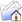LISTSERV Archives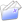PED-EM-L HomePED-EM-L December 1999

Subject:Re: PED-EM-L Digest - 29 Nov 1999 to 30 Nov 1999

From:Martin Herman <[log in to unmask]>

Reply-To:Martin Herman <[log in to unmask]>

Date:Wed, 1 Dec 1999 18:00:14 -0600

Content-Type:text/plain

Parts/Attachments: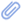text/plain (93 lines)
 ```This is a multi-part message in MIME format.   ------=_NextPart_000_0007_01BF3C25.EAF8E5A0 Content-Type: text/plain;         charset="iso-8859-1" Content-Transfer-Encoding: quoted-printable   Randy is right. If my calculations were typed in wrong. I apologize. It = is true that racemic epi is 2.25% but that means 22.5mg/ml (not = 2.25mg/ml as was stated in the reply).=20 Now half a cc is 11.25 mg of d,l epi, which then would yield about 5.625 = mg l-epi.=20   Still contend that in the more severe bronchiolitics nothing really = helps for long but the l-epi might provide some temporary relief.=20   Marty     ------=_NextPart_000_0007_01BF3C25.EAF8E5A0 Content-Type: text/html;         charset="iso-8859-1" Content-Transfer-Encoding: quoted-printable   =FF=FE<=00!=00D=00O=00C=00T=00Y=00P=00E=00 =00H=00T=00M=00L=00 = =00P=00U=00B=00L=00I=00C=00 = =00"=00-=00/=00/=00W=003=00C=00/=00/=00D=00T=00D=00 =00H=00T=00M=00L=00 = =004=00.=000=00 = =00T=00r=00a=00n=00s=00i=00t=00i=00o=00n=00a=00l=00/=00/=00E=00N=00"=00>=00= =0D=00=0A= =00<=00H=00T=00M=00L=00>=00<=00H=00E=00A=00D=00>=00=0D=00=0A= =00<=00M=00E=00T=00A=00 = =00c=00o=00n=00t=00e=00n=00t=00=3D=00"=00t=00e=00x=00t=00/=00h=00t=00m=00= l=00;=00 = =00c=00h=00a=00r=00s=00e=00t=00=3D=00u=00n=00i=00c=00o=00d=00e=00"=00 = =00h=00t=00t=00p=00-=00e=00q=00u=00i=00v=00=3D=00C=00o=00n=00t=00e=00n=00= t=00-=00T=00y=00p=00e=00>=00=0D=00=0A= =00<=00M=00E=00T=00A=00 = =00c=00o=00n=00t=00e=00n=00t=00=3D=00"=00M=00S=00H=00T=00M=00L=00 = =005=00.=000=000=00.=002=003=001=004=00.=001=000=000=000=00"=00 = =00n=00a=00m=00e=00=3D=00G=00E=00N=00E=00R=00A=00T=00O=00R=00>=00=0D=00=0A= =00<=00S=00T=00Y=00L=00E=00>=00<=00/=00S=00T=00Y=00L=00E=00>=00=0D=00=0A= =00<=00/=00H=00E=00A=00D=00>=00=0D=00=0A= =00<=00B=00O=00D=00Y=00>=00=0D=00=0A= =00<=00D=00I=00V=00>=00<=00F=00O=00N=00T=00 = =00f=00a=00c=00e=00=3D=00A=00r=00i=00a=00l=00 = =00s=00i=00z=00e=00=3D=002=00>=00R=00a=00n=00d=00y=00 =00i=00s=00 = =00r=00i=00g=00h=00t=00.=00 =00I=00f=00 =00m=00y=00 = =00c=00a=00l=00c=00u=00l=00a=00t=00i=00o=00n=00s=00 =00w=00e=00r=00e=00 = =00t=00y=00p=00e=00d=00 =00i=00n=00 =00=0D=00=0A= =00w=00r=00o=00n=00g=00.=00 =00I=00 = =00a=00p=00o=00l=00o=00g=00i=00z=00e=00.=00 =00I=00t=00 =00i=00s=00 = =00t=00r=00u=00e=00 =00t=00h=00a=00t=00 =00r=00a=00c=00e=00m=00i=00c=00 = =00e=00p=00i=00 =00i=00s=00 =002=00.=002=005=00%=00 =00b=00u=00t=00 = =00t=00h=00a=00t=00 =00m=00e=00a=00n=00s=00 =00=0D=00=0A= =00<=00S=00T=00R=00O=00N=00G=00>=002=002=00.=005=00m=00g=00/=00m=00l=00&=00= n=00b=00s=00p=00;=00(=00n=00o=00t=00 =00 = =002=00.=002=005=00m=00g=00/=00m=00l=00 =00a=00s=00 =00w=00a=00s=00 = =00s=00t=00a=00t=00e=00d=00 =00i=00n=00 =00t=00h=00e=00 = =00r=00e=00p=00l=00y=00)=00.=00<=00/=00S=00T=00R=00O=00N=00G=00>=00 = =00=0D=00=0A= =00<=00/=00F=00O=00N=00T=00>=00<=00/=00D=00I=00V=00>=00=0D=00=0A= =00<=00D=00I=00V=00>=00N=00o=00w=00 =00h=00a=00l=00f=00 =00a=00 = =00c=00c=00 =00i=00s=00 =001=001=00.=002=005=00 =00m=00g=00 =00o=00f=00 = =00d=00,=00l=00 =00e=00p=00i=00,=00 =00w=00h=00i=00c=00h=00 = =00t=00h=00e=00n=00 =00w=00o=00u=00l=00d=00 =00y=00i=00e=00l=00d=00 = =00a=00b=00o=00u=00t=00 =005=00.=006=002=005=00 =00m=00g=00 =00=0D=00=0A= =00l=00-=00e=00p=00i=00.=00 =00<=00/=00D=00I=00V=00>=00=0D=00=0A= =00<=00D=00I=00V=00>=00&=00n=00b=00s=00p=00;=00<=00/=00D=00I=00V=00>=00=0D= =00=0A= =00<=00D=00I=00V=00>=00S=00t=00i=00l=00l=00 = =00c=00o=00n=00t=00e=00n=00d=00 =00t=00h=00a=00t=00 =00i=00n=00 = =00t=00h=00e=00 =00m=00o=00r=00e=00 =00s=00e=00v=00e=00r=00e=00 = =00b=00r=00o=00n=00c=00h=00i=00o=00l=00i=00t=00i=00c=00s=00 = =00n=00o=00t=00h=00i=00n=00g=00 =00r=00e=00a=00l=00l=00y=00 = =00h=00e=00l=00p=00s=00 =00=0D=00=0A= =00f=00o=00r=00 =00l=00o=00n=00g=00 =00b=00u=00t=00 =00t=00h=00e=00 = =00l=00-=00e=00p=00i=00 =00m=00i=00g=00h=00t=00 = =00p=00r=00o=00v=00i=00d=00e=00 =00s=00o=00m=00e=00 = =00t=00e=00m=00p=00o=00r=00a=00r=00y=00 =00r=00e=00l=00i=00e=00f=00.=00 = =00<=00/=00D=00I=00V=00>=00=0D=00=0A= =00<=00D=00I=00V=00>=00&=00n=00b=00s=00p=00;=00<=00/=00D=00I=00V=00>=00=0D= =00=0A= =00<=00D=00I=00V=00>=00M=00a=00r=00t=00y=00<=00/=00D=00I=00V=00>=00=0D=00=0A= =00<=00D=00I=00V=00>=00&=00n=00b=00s=00p=00;=00<=00/=00D=00I=00V=00>=00<=00= /=00B=00O=00D=00Y=00>=00<=00/=00H=00T=00M=00L=00>=00=0D=00=0A= =00 ------=_NextPart_000_0007_01BF3C25.EAF8E5A0--   For more information, send mail to [log in to unmask] with the message: info PED-EM-L The URL for the PED-EM-L Web Page is:   http://www.brown.edu/Administration/Emergency_Medicine/ped-em-l.html ```

Advanced Options

#### Options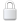Log In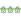Get Password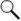Search Archives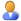Subscribe or Unsubscribe